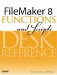Category: Trigonometric

Parameters:

angleInDegreesA number representing an angle measured in degrees.

Data type returned: Number

Description:

The Radians() function converts an angle measured in degrees into an angle measured in radians.

The trigonometric functions Sin(), Cos(), and Tan() all take an angle measured in radians as their parameter. To find, say, the cosine of a 180° angle, you could use the formula Cos (Radians (180)). There are 2xPi radians in 360°.

Examples:

Function

Results

Returns 1.0471975511965977 (= Pi/3).

Returns 3.1415926535897932 (= Pi).FileMaker 8 Functions and Scripts Desk Reference
ISBN: 0789735113
EAN: 2147483647
Year: 2004
Pages: 352